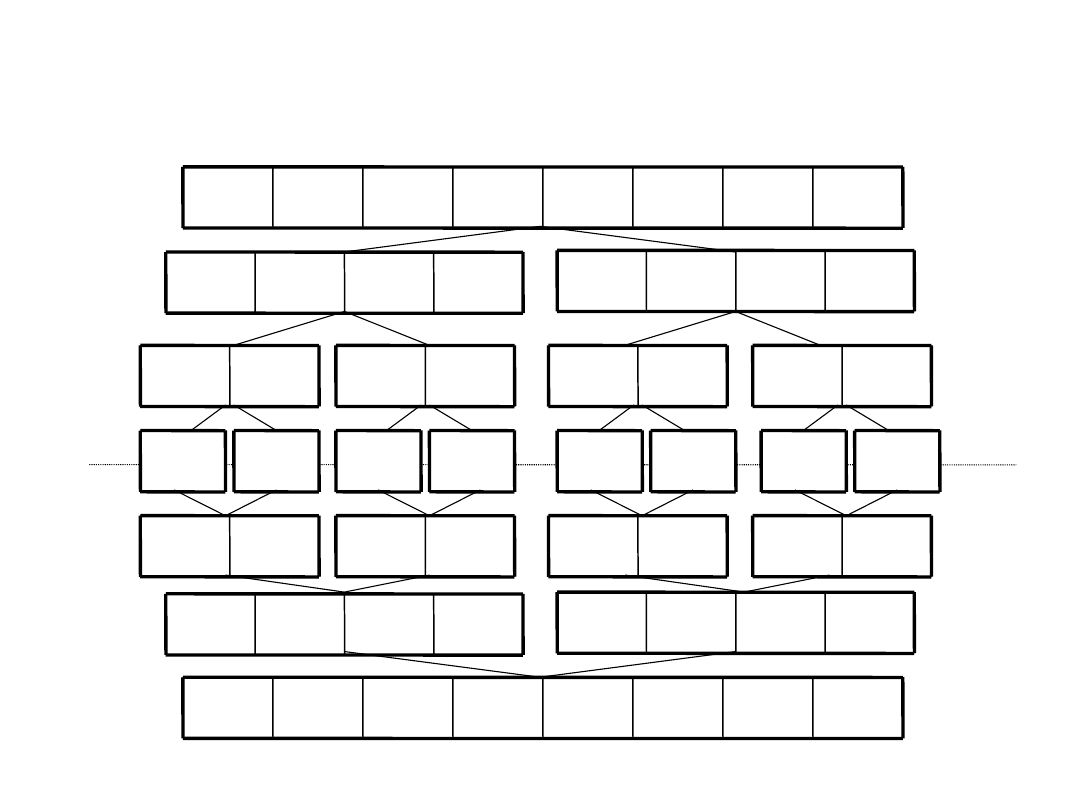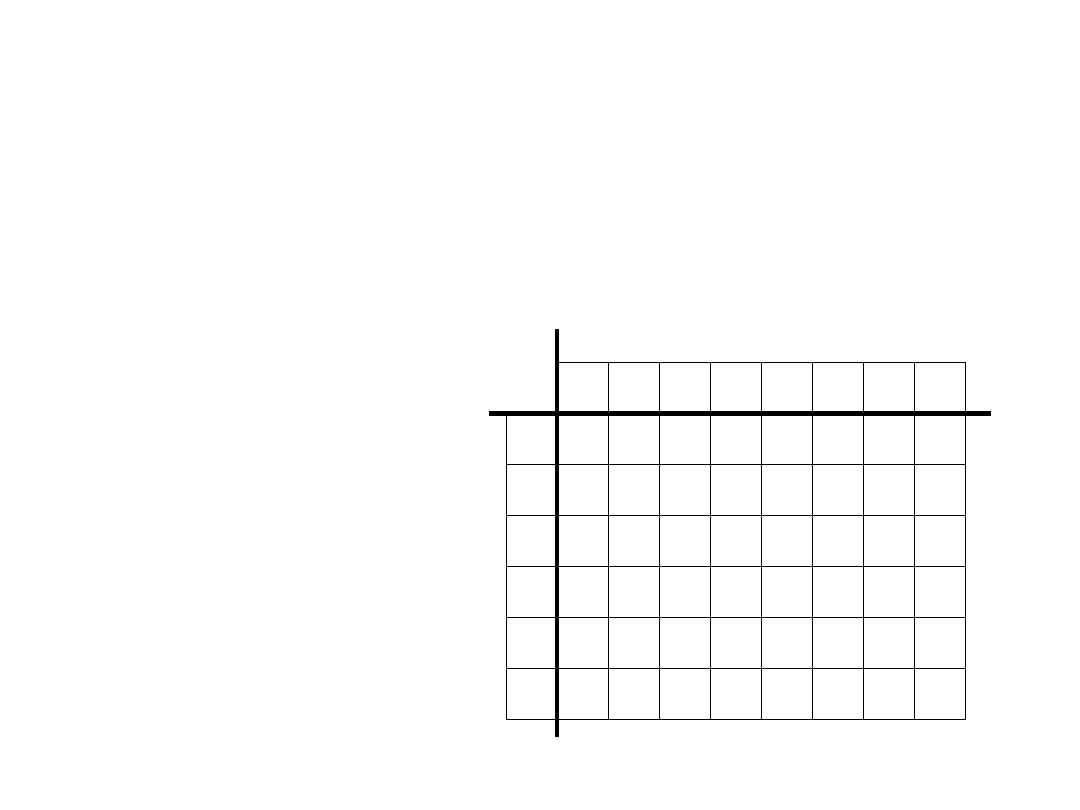## DSaA W04 Techniques id 143853 NieznanyPobierz cały dokument DSaA W04 Techniques id 143853 Nieznany .pdf Rozmiar 295,8 KB1

DSaA 2012/2013

Fundamental Techniques

Data Structures and Algorithms2

DSaA 2012/2013

Fundamental Techniques

Fundamental techniques:
• Divide and conquer
• Dynaming programming
• Greedy algorithm3

DSaA 2012/2013

Divide and conquer

• A problem input (instance) is divided according to some

criteria into a set of smaller inputs to the same
problem. The problem is then solved for each of these
smaller
inputs, either recursively by further division into
smaller inputs or by invoking an ad hoc or a priori
solution. Finally, the solution for the original input is
obtained by expressing it in some form as a
combination of the solution
for these smaller inputs.

• Ad hoc solution are often invoked when the input size is

smaller than some preassigned threshold value.

• Subproblems are independent!4

DSaA 2012/2013

Divide and conquer

procedure Divide_and_conquer(I,J)
Input: I (an input to the given problem)
output: J (a solution to the given problem

corresponding to the input I)

if I is_Known then
assign the a priori or ad hoc solution for I to J
else
Divide(I, I

1

,…,I

m

) // m may depend on the input I

for i=1 to m do
Divide_and_conquer(I

i

,J

i

)

endfor
Combine(J

1

,…,J

m

,J)

endif
end
Divide_and_conquer5

DSaA 2012/2013

Divide and conquer

– mergesort

Merge-sort:

1. Divide the input part of table into two equal (equally

likely) parts A and B

2. Sort part A

3. Sort part B

4. Merge parts A and B, knowing that this part are

sorted

Stop the recurrence if size of an input part is
equal 1. The table with only one element is
always sorted.6

DSaA 2012/2013

Mergesort

– an example

2

4

15

1

3

20

6

5

3

20

6

5

2

4

15

1

6

5

3

20

15

1

2

4

5

6

20

3

1

15

4

2

6

5

20

3

15

1

4

2

20

6

5

3

15

4

2

1

20

15

6

5

4

3

2

1

recu

ren

cy

return

from

recuren

cy7

DSaA 2012/2013

Dynaming programming

Dynamic programming is similar to divide-and-

conquer in the sense, that it is based on
recursive division of problem instances into
smaller or simpler problem instances.. However,
whereas divide-and-conquer algorithms often
use a top-down resolution method, DP
algorithms invariably proceed by solving all the
simplest
problem instances before combining
then into more complicated problem instances
in a bottom-up fashion.

• Unlike in divide-and-conquer the subproblems

share a subsubproblems8

DSaA 2012/2013

Dynaming programming

– LCS

Longest common subsequence (LCS)
• Let A be a sequence A=a

0

a

1

…a

n-1

. A

subsequence of A is a sequence

where

• example „samples” -> „sms”,”ss”, „mp”
• If we have two sequence A=a

0

a

1

…a

n-1

and

B=b

0

b

1

…b

m-1

we a looking for a longest

common substring C, which is a
subsequence of A and B.

1

1

0

k

i

i

i

a

a

a

T

n

i

i

i

k

1

1

0

09

DSaA 2012/2013

LCS

• In real we will compute the length of the LCS. Let LCS[i,j]

will be the length of longest common subsequence of
sequences

A’=a

0

a

1

…a

i-1

and

B’=b

0

b

1

…b

j-1

otherwise

j

i

LCS

j

i

LCS

b

a

if

j

i

LCS

j

or

i

if

j

i

LCS

j

i

])

,

1

[

],

1

,

[

max(

1

]

1

,

1

[

0

0

0

]

,

[

1

1

• We can compute the equation recursively, but better way

is to use an array and compute the values of LCS row by
row from 0 to m-1 and for every row cells from 0 to n-110

DSaA 2012/2013

LCS

– an example

• A=„abbaa”
• B=„bababab”
• n=5
• m=7

• LCS[n,m]=

a

b

a

b

a

b

b

0

2

1

4

3

6

5

7

0

0

0

0

0

0

0

0

0

1

0

1

1

1

1

1

0

1

1

a

b

b

a

a

0

1

2

3

4

511

LCS

– an example

DSaA 2012/2013

• A=„abbaa”
• B=„bababab”
• n=5
• m=7

• LCS[n,m]=4

a

b

a

b

a

b

b

0

2

1

4

3

6

5

7

0

0

0

0

0

0

0

0

0

1

0

1

1

1

1

1

0

1

1

2

2

2

2

2

0

1

1

2

2

3

3

3

0

2

1

3

2

4

3

4

0

2

1

3

2

4

3

4

a

b

b

a

a

0

1

2

3

4

512

DSaA 2012/2013

Greedy algorithm

The greedy method for solving optimization problems follows the

philosophy of greedily maximizing (or minimizing) short-term gain
and hoping for the best without regard to long term consequences.

• Making decision based on optimizing short-term gain may not lead

to solution that is optimal. So that you always need to prove that
greedy solutions are indeed optimal

Advance: Algorithms based on the greedy method are usually very

simple, easy to code, and efficient

Disadvance: when we uses the greedy method in algorithm to solve

a problem, we often end up with less-than-optimal result.

Advance: for some important problem the greedy method does

yield optimal results (it is proved)!

Advance: in some important problems, the greedy method yields

results that are not optimal but in some sense are good
approximations
to optimal results.13

DSaA 2012/2013

Greedy algorithm

procedure Greedy(S,Solution)
input: S (base set) // it is assumed that there is an associated objective

// function f defined on (possibly ordered) subsets of S

output: Solution (an ordered subset of S that potentially optimizes
the objective function f, or a message that Greedy
doesn’t even produce a solution, optimal or not)
PartialSolution = Ø // initialize the partial solution to be empty
R=S
while PartialSolution is not a solution and R!=0 do
x=GreedySelect(R)
R=R\{x}
if PartialSolution U {x} is feasible then
PartialSolution= PartialSolution

{x}

endif
endwhile
if PartialSolution is a solution then
Solution=PartialSolution
else
write(„Greedy fails to produce a solution”)
endif
end
Greedy14

DSaA 2012/2013

Greedy algorithm

– making change

Making change - suppose we have just

purchased something and the salesperson
wishes to give back exact change using the
fewest number of coins. We assume that there
is a sufficient amount of coins to make a change
in any manner whatsoever.

• A greedy algorithm for making changes uses as

many coins of the largest denomination as
possible, then uses as many coins of the next
largest denomination, and so forth.15

DSaA 2012/2013

Making change

– an example(1)

possible coins: 1gr, 2gr, 5gr, 10gr, 20gr, 50gr
• total change=97gr
• change 50gr, rest=47gr
• change 20gr, rest=27gr
• change 20gr, rest=7gr
• change 5gr, rest=2gr
• change 2gr, rest=0gr
• number of coins = 516

DSaA 2012/2013

Making change

– an example(2)

possible coins: 1gr, 4gr, 5gr, 10gr, 40gr, 50gr
• total change=88gr
• change 50gr, rest=38gr
• change 10gr, rest=28gr
• change 10gr, rest=18gr
• change 10gr, rest=8gr
• change 5gr, rest=3gr
• change 1gr, rest=2gr
• change 1gr, rest=1gr
• change 1gr, rest=0gr
• number of coins = 8

• the best solution 88gr=40gr+40gr+4gr+4gr, number of coins = 4
By extension the short-term of gain we can improve the algorithm to

be correct.17

Example problems

http://livearchive.onlinejudge.org/

->Browse Problems

ICPC Archive Volumes

2487 - Lollies
2122 - Recognizing S Expressions
2535 - Magnificent Meatballs
3390 - Pascal's Travels

DSaA 2012/2013Pobierz cały dokument DSaA W04 Techniques id 143853 Nieznany .pdf Rozmiar 295,8 KB

Wyszukiwarka

Podobne podstrony:
98 o dozorze technicznym id 487 Nieznany (2)
5 3 pl warunki techniczne id 39 Nieznany (2)
MM ETK W04 zmiennestanu id 3442 Nieznany
al1 w04 zima2011 id 54566 Nieznany (2)
Mikrogeneracja Technika id 3014 Nieznany
opis techniczny id 400099 Nieznany
anl1 w04 zima2012 id 65275 Nieznany (2)
anl1 w04 lato2009 id 65274 Nieznany (2)
pismo techniczne B id 359164 Nieznany
Opis techniczny 5 id 337061 Nieznany
M W04 57 id 274844 Nieznany
newsy technika id 317929 Nieznany
98 o dozorze technicznym id 487 Nieznany (2)
gs w04 id 197501 Nieznany
Opis techniczny dachu id 337093 Nieznany
NOWE TECHNIKI W SYS VSAD id 32 Nieznany
Metodyka nauczania techniki id Nieznany
krs form w04 id 251003 Nieznany

więcej podobnych podstron